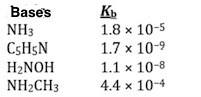Chemistry Practice Problems Conjugate Acids and Bases Practice Problems Solution: Using the data in the table, which of the conjugat...

🤓 Based on our data, we think this question is relevant for Professor Rightmire's class at UNR.

# Solution: Using the data in the table, which of the conjugate acids below is the weakest acid? A) NH 3CH 3 + B) H 3NOH + C) C 5H 5NH + D) NH 4 + E) NH 4 + and NH 3CH 3 +

###### Problem

Using the data in the table, which of the conjugate acids below is the weakest acid?

A) NH 3CH +

B) H 3NOH +

C) C 55NH +

D) NH +

E) NH + and NH 3CH +Conjugate Acids and Bases

Conjugate Acids and Bases

#### Q. Identify the conjugate base in the reaction: HClO2 (aq) + H2O (l) → H3O+(aq) + ClO2-(aq) (a) HClO2 (b) H2O (c) H3O+ (d) ClO2-

Solved • Wed May 31 2017 04:18:01 GMT-0400 (EDT)

Conjugate Acids and Bases

#### Q. Which of the following statement(s) is/are correct.i. OH- is the conjugate acid of H 2O.ii. C3H7NH3+ is the conjugate acid of C3H7NH2.iii. HPO42- is t...

Solved • Fri Apr 28 2017 15:27:08 GMT-0400 (EDT)

Conjugate Acids and Bases

#### Q. What is the conjugate base of HSO 4– in the reaction below? CO32– + HSO4–  ⇌  HCO3– + SO42– A)  HSO4–             B)  CO32–                 C)  OH–...

Solved • Wed Mar 22 2017 16:29:48 GMT-0400 (EDT)

Conjugate Acids and Bases

#### Q. What is the conjugate acid of CO 32– in the reactionCO32– + HSO4- ⇌ HCO3– + SO42–A) HCO3–             B) HSO4–                C) OH–                D)...

Solved • Wed Mar 22 2017 16:16:55 GMT-0400 (EDT)